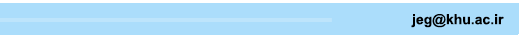[Home ] [Archive]   [ فارسی ]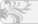Main About Current Issue Archive Search Submit Contact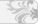Home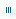Journal InformationArticles archiveFor AuthorsFor ReviewersRegistrationContact usSite FacilitiesSearch in website
 Search the articlesSearch the site Advanced SearchEmblem Journal concessionaire  Kharazmi University Managing director Seyed Mahmoud Fatemi Aghda, Professor Editor-In-Chief Ali Ghanbari, Professor Director Ali Noorzad, Associate Professor Manager Fatemeh Torabi ISSN: 2228-6837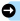Search published articles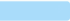Showing 4 results for Mokhtari

Aref Alipour, Mojtaba Mokhtarian,
Volume 13, Issue 4 (Vol. 13, No. 4 2020)
Abstract

Introduction
The main objective of this contribution is to focus on the portion of the comminution process which deals with the prediction of the energy consumption due to the comminution portion of the milling processes.
The comminution energy in mineral processing and cement industry is usually determined by empirical Bond Work Index (BWI), regardless of the mechanical properties of a rock. The BWI is a measure of ore resistance against grinding and is determined by using the Bond grindability test. Determining the BWI value is quite complicated and time consuming. Its value constitutes ore characteristic and is used for industrial commination plants designing and optimization. The BWI is deﬁned as the calculated speciﬁc energy (kW h/t) applied in reducing material of inﬁnite particle size to 80% passing 100 µm. The higher the value for BWI, the more energy is required to grind a material in a ball mill. The energy consumed in the process of comminution depends on both the mechanism of comminution and the mechanical properties of the materials being ground. It is interesting to study the effect of the essential ones of these properties on the energy efficiency of grinding process.
Material and methods
Several attempts have been made to obtain and optimize the comminution energy. An efficient Response Surface Method, (RSM)-based method for the BWI approximate value determination, which is based on physico-mechanical tests, is presented in this paper.
BWI and some physico-mechanical tests on 8 typical rock samples and its correlation are studied; it would be beneficial to examine this relation based on physical concept. The database including Uniaxial Compressive Strength (UCS), Abrasion (AT), Hardness (HT) and Modulus of Elasticity (ME) are assembled by collecting data from Haffez experiments.
Results and discussion
The determination of the BWI from RSM- based multivariate model is almost matched with measured Bond’s work index. As a result of analysis the best equation obtained from RSM-based model is formulized in Equation 1:
(1)
Standard statistical evaluation criteria are used to evaluate the performances of predictive models.
Conclusion
The performance of the estimator models can be controlled by R2, VAF, RMSE, MAPE, VARE and MEDAE. The RSM- based model with higher VAF as well as lower RMSE, MAPE, VARE, MEDAE shows better performance in comparison to the Haffez single-variable models. AT and ME have the greatest effect on the value of BWI; and also HT has the least impact../files/site1/files/134/6.pdf
Maryam Mokhtari, Kazem Barkhordari, Saeid Abbasi Karafshani,
Volume 13, Issue 5 (English article specials 2020)
Abstract

In recent years, with the growing use of the nailing method for stabilizing excavation walls, there has been a need for a comprehensive investigation of the behavior of this method. In the  previous studies, the behavior of nailed walls has been investigated in static and dynamic states and under different conditions. However, due to the different feature of near-field ground motions, it is  necessary to study the effect of these motions on the behavior of the nailed walls. Near-fault ground motion is significantly affected by the earthquake record direction and the rupture mechanism. So, in this study, to compare the effects of near-field and far-field ground motions, a two-dimensional (2D) soil- nailed wall was considered. PLAXIS 2D was used for the modeling of the soil-nailed wall system. An excavation with a dimension of 10 meters in height was taken into the account. In this study, 10 records (Five fault-normal near-field ground motion records and five far-field ground motion records), were recorded  on the rock and  applied to the model. These ground motion records were derived from the near-fault ground motion record set used by Baker. These records were scaled to the Peak Ground Acceleration (PGA) of 0.35g and then applied to the bottom of the finite element models. Mohr-Coulomb model was then used to describe the soil behavior, and Elasto-plastic model was employed for the nails. A damping ratio of 0.05 was considered at the fundamental periods of the soil layer. The results showed that the  generated values of bending moment, shear force and axial force in nails under the effect of the near-fault ground motions were  more than those in the far-ault ground motions. These values were  almost equal to 23% for the maximum bending moment, 30% for the  shear force,  and 22% for the axial force. The created displacement under the effect of near-fault ground motions was  more than that in the far-fault since a higher energy was  applied to the model in the near-field ground motions during a short time (pulse-like ground motions). In contrast, in the far-fault ground motions, due to the more uniform distribution of energy during the record, such pulse-like displacements were not observed in the system response. Increasing in nail length and soil densification, decreases the displacement of the soil-nailed wall but does not change the general behavior of the soil under the effect of near-field ground motions. Based on the obtained results, for a constant PGA, there were  positive correlations between the values of the  maximum displacement on the top of the wall and  the PGV values of near-fault ground motion records. However, the mentioned correlations were  not observed in the case of far-fault ground motions.

Saeed Mahdavi, Mehrnosh Haghighat, Maryam Mokhtari,
Volume 14, Issue 1 (5-2020)
Abstract

Introduction
Material and Methods
The dam site is placed approximately at a distance of 2.7 km from the intersection of Koohrange and Beheshtabad river. In accordance with geological studies, the rocks in the site could be categorized in four units combined of Dolomite, Dolomitic Limestone, Limestone, Marl and Marly Limestone. Applying empirical equation the rock mass modulus of dam abutments is evaluated based on the laboratory test results and rock mass engineering classification systems. In addition, ASTM D4394 is applied to investigate the results of ten plate loading tests which are executed in the right and left abutments. To interpret the plate loading test results in the right abutment, a three-dimensional Fast Lagrange Analysis of Continuum (FLAC3D) model is developed.
Result and Discussion
To process the numerical simulation results, back analysis as a data processing tool is used. In this approach, the input parameters of numerical model will be changed in the way that the measured quantities by extensometers at the monitoring points are almost equal with the computed ones via numerical model at the corresponding points. Based on the sensitivity analysis carried out on the Mohr-Coulomb failure criterion parameters, the friction coefficient and cohesion variation do not affect the displacements calculated via numerical simulation as the more portion of gallery displacements are elastic. The error function is minimum when the rock mass modulus is 12 GPa and the horizontal to vertical stress ratio (K0) is equal to 0.5. The evaluated rock mass modulus based on the numerical simulation is two times lower than corresponding one evaluated applying empirical equation as a result of empirical equation uncertainty. Consideration of stress decrement under loading plate shows lower level of stress decrement under loading plate in ASTM D4394 compared to numerical simulation. This is why, the rock mass modulus, calculated based on ASTM D4394, increases dramatically by getting distance from the loading plate.
Conclusion
The empirical methods estimating the modulus of deformation based on rock mass classification systems tend to evaluate large value of modulus especially for the weak massive rocks.
As a result of galleries dimensions and semi-infinite boundary condition assumed in ASTM D4394, the calculated rock mass modulus increases dramatically by getting distance from loading plate. Therefore, the numerical simulation was applied to process the plate loading test results. A new normalized error function was developed based on measured displacements and the rock mass modulus in the right abutment was determined 12 GPa which is very lower than the calculated value using ASTM D 4394. Also, as a result of numerical simulation, the rock mass is uniform. The stress increment perpendicular to the loading plate was calculated applying numerical simulation which is 0-90 percent lower than those suggested by ASTM D 4394.

Maryam Mokhtari,
Volume 16, Issue 1 (10-2022)
Abstract

In geotechnical engineering, rock mechanics and engineering geology, depending on the project design, uniaxial strength and static Youngchr('39')s modulus of rocks are of vital importance. The direct determination of the aforementioned parameters in the laboratory, however, requires intact and high-quality cores and preparation of their specimens have some limitations. Moreover, performing these tests is time-consuming and costly. Therefore, in this study, it was tried to precisely predict the desirable parameters using physical characteristics and ultrasonic tests. To do so, two methods, i.e. principal components regression and support vector regression, were employed. The parameters used in modelling included density, P- wave velocity, dynamic Poisson’s ratio and porosity. Accordingly, the experimental results conducted on 115 limestone rock samples, including uniaxial compressive and ultrasonic tests, were used and the desired parameters in the modelling were extracted using the laboratory results. By means of correlation coefficient (R2), normalized mean square error (NMSE) and Mean absolute error (MAE), the developed models were validated and their accuracy were evaluated. The obtained results showed that both methods could estimate the target parameters with high accuracy. In support vector regression, Particle Swarm Optimization method was used for determining optimal values of box constraint mode and epsilon mode, and the modelling was conducted using four kernel functions, including linear, quadratic, cubic and Gaussian. Here, the quadratic kernel function yielded the best result for UCS and cubic kernel function yielded the best result for Es. In addition, comparing the results of the principal components regression and the support vector regression indicated that the latter outperformed the former.

 Page 1 from 1